# Aggregating Average Treatment Effects with baggr

#### 2023-03-22

This vignette is written for baggr users who want to learn about (Bayesian) meta-analysis concepts and analyse data on typically continuous variables. If you are looking for information that is more specific to binary data, please read vignette("baggr_binary"). This article - like the package itself - is still under construction. We encourage your feedback.

baggr (pronounced “bagger” or “badger” and short for Bayesian Aggregator) is a package for aggregating evidence on causal effects measured in several separate and different instances. These instances may be different studies, groups, locations or “sites” however conceptualised. We refer to these separate pieces of evidence as “groups” for the remainder of this vignette When each group is a study, the model is that of meta-analysis, but aggregation of evidence is not limited to this case.

One of the most basic objects of interest is the average treatment effect (ATE), the difference in the mean outcome in treatment and control groups; for more information see work by Rubin (1974). In meta-analysis we are often interested in the average of this average effect across groups, estimated using all the evidence from all the groups. Consider the case where the evidence in each study or group is generated by comparing the outcomes of treatment and control samples in a randomized experiment. We will ignore any covariate information at the individual or group level for now.

Consider some outcome of interest $$y_{ik}$$ such as consumption, income or health outcomes for a household or individual $$i = 1,2,...N_k$$ in study group $$k = 1,2....K$$. Let $$Y_k$$ denote the $$N_k$$-length vector of observed outcomes from group $$k$$. Denote the binary indicator of treatment status by $$T_{ik}$$, and denote by $$T_k$$ the $$N_k$$-length vector of all treatment status indicators from group $$k$$.

Suppose that $$y_{ik}$$ varies randomly around its mean $$\mu_k + \tau_k T_i$$. In this setting $$\tau_k$$ is the treatment effect in group $$k$$. The random variation in $$y_{ik}$$ may be the result of sampling variation or measurement error, as in the Rubin (1981) model, or it may be the result of unmodelled heterogeneity or uncertainty in outcomes for individuals within the group. Allow the variance of the outcome variable $$y_{ik}$$ to vary across sites, so $$\sigma_{y_k}^2$$ may differ across $$k$$.

## Data inputs: reported effects or full individual-level data sets

For average effects aggregation, baggr allows 3 types of data inputs. The user may supply, within a data frame environment, any of the following:

1. A set of estimated treatment effects $$\{\hat{\tau_k}\}_{k=1}^{K}$$ and their standard errors $$\{\hat{se_k}\}_{k=1}^{K}$$ from each study. This should be formatted as two column vectors of length $$K$$ within the data frame, where $$\hat{\tau_k}$$ is the $$k$$-th entry of the treatment effect vector and $$\hat{se_k}$$ is the $$k$$-th entry of the standard errors vector. Columns should be named "tau" and "se". Model will be "rubin" (see below).

2. A set of control group means and estimated treatment effects $$\{\hat{\mu_k},\hat{\tau_k}\}_{k=1}^{K}$$, as well as the standard errors for both $$\{\hat{se}_{\mu k}, \hat{se}_{\tau k}\}_{k=1}^{K}$$, for each study site This should be formatted as four vectors of length $$K$$ within the data frame, analogous to the above. Columns should be names "mu", "tau", "se.mu", "se.tau". Model will be "mutau" (see below).

3. The full data sets from all the original studies $$\{Y_k, T_k\}_{k=1}^{K}$$. This should be formatted as three vectors of length $$\sum_{k=1}^K N_{k}$$, which we recommend naming "outcome", "treatment", "group" (for site indicators), but the names can also be specified when calling baggr() function. Model will be "rubin_full" (see below).

As an example of an individual-level data set we include in data frames microcredit and microcredit_simplified. The former contains all the microcredit outcome data used in Meager (2019), standardized to USD PPP in 2009 terms per two weeks (a time period is necessary as these are flow variables). It therefore contains NAs and other undesirable features, to allow the user to see how baggr handles these common data issues. The data set microcredit_simplified has these issues cleaned up and contains only one outcome of interest, consumer durables spending.

baggr also has a function that automatically produces summary data from full data sets, in case one wishes to run the comparatively faster summary-data models. The prepare_ma() function applied to a dataframe with columns named "group", "outcome", and "treatment" automatically estimates the control group means, treatment effects, and associated standard errors for each group using an Ordinary Least Squares regression. The resulting output is already formatted as a valid input to the baggr() command itself:

prepare_ma(microcredit_simplified, outcome = "consumption")
#>   group       mu       tau     se.mu    se.tau n.mu n.tau
#> 1     1 303.6065  5.510755  2.559897  4.101140 8298  8262
#> 2     2 280.0887 50.449393 11.141075 22.156317  260   701
#> 3     3 196.4215 -5.171338 14.432604 19.266339  444   551
#> 4     4 276.2791  4.641604  3.730907  5.451094 3248  3579
#> 5     5 327.5246 -2.935731  4.027768  6.022955 2771  2716

## ATE aggregation models in baggr

baggr currently contains two different models suitable for aggregating sets of average treatment effects. Consider first the evidence aggregation model from Rubin (1981), discussed extensively in Chapter 5 of Gelman et al. (2013); the model consists of a hierarchical likelihood as follows:

\begin{equation} \begin{aligned} \hat{\tau_k} &\sim N(\tau_k, \hat{se_k}^2) \; \forall \; k \\ \tau_k &\sim N(\tau, \sigma_{\tau}^2) \; \forall \; k . \end{aligned} \label{rubin model} \end{equation}

The motivation for this model structure is discussed in detail in the sources above and in Meager (2019). To complete the Bayesian model, we now need priors. baggr has a set of default priors for each model (adjusted to data), as well as allowing the user to specify her own priors if desired. In the Rubin model, baggr’s default priors on the hyper-parameters are as follows:

• For $$\tau$$, the prior is $$\mathcal{N}(0, 100)$$. This is a very weak prior which does little regularization as a default, centered at zero. It assumes that causal effects should not be thought of as large unless data contains evidence to the contrary (a “hand-wavey” form of Occam’s Razor).
• For $$\sigma_{\tau}$$ the prior is $$\textrm{Uniform}(0,10\tilde{\sigma})$$, where $$\tilde{\sigma}$$ is a naive standard deviance estimator for $$\{\hat{\tau_k}\}_{k=1}^{K}$$ (generated by the R command sd()).

In case you also have data on the control groups’ mean outcomes and the uncertainty on those,you can augment the Rubin (1981) model to incorporate that information. Following Meager (2019), if one has access to the estimated control means $$\{\hat{\mu_k}\}^K_{k=1}$$ and their standard errors $$\{\hat{se}_{\mu k}\}^K_{k=1}$$, one can fit a joint Gaussian model on the pairs $$\{\hat{\mu_k},\hat{\tau_k}\}_{k=1}^{K}$$:

\begin{equation} \begin{aligned} \hat{\tau_k} &\sim N(\tau_k, \hat{se_{\tau k}}^2) \; \forall \; k \\ \hat{\mu_k} &\sim N(\mu_k, \hat{se_{\mu k}}^2) \; \forall \; k \\ \left( \begin{array}{c} \mu_{k}\\ \tau_{k} \end{array} \right) &\sim N\left( \left( \begin{array}{c} \mu\\ \tau \end{array} \right), V \right) \; \text{where} \;V = \left[ \begin{array}{cc} \sigma^2_{\mu} & \sigma_{\tau\mu} \\ \sigma_{\tau\mu} & \sigma_{\tau}^2 \end{array} \right]\forall \; k. \\ \end{aligned} \label{full data model} \end{equation}

In baggr this model is referred to as "mutau".

If you have only few groups, the priors on $$V$$ will need to be relatively strong to avoid overfitting. See Meager (2019) for more discussion of this issue in particular, or see the Stan Manual on hierarchical priors. The default priors are as follows:

• $$V$$ is decomposed into a correlation matrix $$\Omega$$, which receives an LKJCorr(3) prior regularizing it towards independence, and a scale parameter $$\theta$$ which receives a $$\textrm{Cauchy}(0,s)$$ prior, with $$s$$ set to either 10 times the empirical SD of $$\mu$$ of of $$\tau$$, whichever is higher.
• The default prior for $$(\mu, \tau)$$ is bivariate Gaussian, with zero means and a diagonal covariance matrix with the diagonal (variances) set to 100 times the empirical maximum of $$\mu$$ and $$\tau$$.

## Models, their inputs, likelihood and priors: a summary table

Model (name in baggr) Input columns Level-1 likelihood Level-2 likelihood Default priors
summary data “Rubin” ("rubin") tau and se $$\hat{\tau_k} \sim N(\tau_k, \hat{se_k}^2)$$ $$\tau_k \sim N(\tau, \sigma_{\tau}^2)$$ $$\tau \sim \mathcal{N}(0, 100)$$, $$\sigma_{\tau} \sim \mathcal{U}(0, 10\tilde{\sigma})$$
$$\mu$$ and $$\tau$$” ("mutau") tau, mu, se.tau, se.mu $$\hat{\tau_k} \sim N(\tau_k, \hat{se_{\tau,k}}^2)$$, $$\hat{\mu_k} \sim N(\mu_k, \hat{se_{\mu,k}}^2)$$ $$\pmatrix{\mu_k \\ \tau_k} \sim N(\pmatrix{\mu \\ \tau}, V)$$ $$V = \theta \Omega \theta'$$ where $$\theta \sim Cauchy(0,10)$$, $$\Omega \sim LKJ(3)$$, $$\pmatrix{\mu \\ \tau} \sim N(0,100^2Id_2 )$$
full data “Rubin” ("rubin_full") outcome, treatment, group Same as for “$$\mu$$ and $$\tau$$ Same as for “$$\mu$$ and $$\tau$$ Same as for “$$\mu$$ and $$\tau$$
Logit ("logit") outcome, treatment, group See vignette("baggr_binary")

Where inputs are individual-level data, i.e. outcome, treatment, group, you can specify column names in as arguments to baggr() function.

## Prior choice in baggr

In the “Rubin” and “$$\mu$$ and $$\tau$$” models, the user can specify custom priors beyond the defaults using the prior arguments. These prior arguments are subdivided into 3 categories:

• Priors for the hypermean, the average effect across groups, such as $$\tau$$ or the vector $$(\mu, \tau)$$. This is denoted using the prior_hypermean argument in baggr.

• Priors for the hyper-standard-deviations $$\sigma_{\tau}$$, the standard deviation of effects across groups. This also refers to the prior on $$\theta$$, the scale parameter of the hyper-variance-covariance matrix $$V$$ in the”$$\mu$$ and $$\tau$$” model. This type of parameter is denoted prior_hypersd in baggr.

• Priors on hypercorrelations between parameters that may co-vary across the groups. When working with multi-dimensional parameters at the upper level of the model (aka performing multivariate shrinkage), this is the correlation in the distribution of the different parameters across the groups. For example, in the “$$\mu$$ and $$\tau$$” model this parameter is the correlation matrix $$\Omega$$ that governs the hyper-variance-covariance matrix $$V$$. This type of parameter is denoted prior_hypercor in baggr.

The possible prior distributions we allow for in the current version are:

• For prior_hypermean we allow "normal", "uniform", "cauchy", "lognormal", and (generalised) "student_t" with any parameter values which are logically possible given support constraints (e.g. user cannot specify a negative variance on a normal distribution or a negative scale on a Cauchy) When the model has a vector hypermean, “$$\mu$$ and $$\tau$$” model, baggr applies the given prior to all the elements of the vector independently. If the user wishes to specify a prior dependence between the components, one can supply prior_hypermean with a multinormal argument (see ?priors for details).
• For prior_hypersd we allow "normal" and "uniform" with any parameter values which are logically possible given support constraints (as above).
• For prior_hypercor, which is only necessary when there is a multivariate shrinkage operation (as in the “$$\mu$$ and $$\tau$$” model) we allow an LKJ correlation prior ("lkj") with any parameter values which are logically possible given support constraints. For further details of this strategy see Meager (2019).

Notation for priors is “plain-text”, in that you can write the distributions as normal(), uniform() etc. See ?priors for details, or continue to the example below with the Rubin model.

## Running the Rubin Model in baggr

To demonstrate the Rubin model in baggr, consider the 8 schools example from Rubin (1981). In this dataset, 8 schools in the United States performed similar randomized experiments to estimate the causal effect of an SAT tutoring program on learning outcomes. Reported treatment effects and their standard errors are included in baggr as a data frame:

schools
#>      group tau se
#> 1 School A  28 15
#> 2 School B   8 10
#> 3 School C  -3 16
#> 4 School D   7 11
#> 5 School E  -1  9
#> 6 School F   1 11
#> 7 School G  18 10
#> 8 School H  12 18

To fit the model in baggr (having followed the installation instructions and loaded the package):

baggr_schools <- baggr(schools, model = "rubin", pooling = "partial")

This creates a baggr class object, and you can access the underlying stanfit object by calling baggr_schools$fit. If you don’t change the default priors, then baggr will print a message informing you of the priors it has chosen. Printing baggr_schools returns a summary of the posterior inference. First baggr records the model type and the pooling regime chosen by the user or implemented by default. Second, baggr returns inference on the aggregate treatment effect $$\tau$$ by reporting its posterior mean and 95% uncertainty interval, and similar inference on the hyper-SD $$\sigma_{\tau}$$. Lastly, it prints the “updated” inference on each of the groups’ treatment effects, displaying their new posterior means, standard deviations and pooling factors (see below). print(baggr_schools) #> Model type: Rubin model with aggregate data #> Pooling of effects: partial #> #> Aggregate treatment effect (on mean), 8 groups: #> Hypermean (tau) = 8.0 with 95% interval -1.9 to 18.7 #> Hyper-SD (sigma_tau) = 6.43 with 95% interval 0.22 to 20.42 #> Total pooling (1 - I^2) = 0.77 with 95% interval 0.25 to 1.00 #> #> Group-specific treatment effects: #> mean sd 2.5% 50% 97.5% pooling #> School A 11.1 8.5 -3.1 10.0 31 0.83 #> School B 7.9 6.3 -4.9 7.8 21 0.73 #> School C 6.3 7.7 -11.0 6.8 21 0.84 #> School D 7.6 6.4 -5.3 7.7 21 0.75 #> School E 5.1 6.4 -8.9 5.7 16 0.70 #> School F 6.2 6.7 -9.2 6.7 19 0.75 #> School G 10.5 6.7 -1.2 9.9 26 0.73 #> School H 8.4 7.9 -7.5 8.2 26 0.87 ### Choosing priors It is possible to fit the Rubin model without specifying any priors, in which case the user is notified about the automatic prior choice that baggr performs. But the priors can also be easily customised by using prior_ arguments to baggr(). The Rubin model performs univariate shrinkage so we will not need to specify a hyper-correlation prior, but we can specify some custom priors on the hyper-mean and hyper-sd. If desired, the user can specify only some priors as custom distributions - the rest will be chosen automatically and the user will be notified of this in the output. Consider changing both as an example, say placing a Normal prior with mean -5 and standard deviation 10 on our hypermean $$\tau$$, as well as placing a uniform prior with lower bound 0 and upper bound 5 on our hyper-standard-deviation $$\sigma_{\tau}$$. Thus, what is expressed mathematically as $$\tau \sim N(-5,10)$$ and $$\sigma_{\tau} \sim U[0,5]$$ is expressed in baggr as follows: baggr(schools, "rubin", prior_hypermean = normal(-5, 10), prior_hypersd = uniform(0, 5)) It is also possible to pass your custom priors as a list to baggr, as follows: custom_priors <- list( hypermean = cauchy(0,25), hypersd = normal(0,30)) baggr(schools, "rubin", pooling = "partial", prior = custom_priors) Note that the Rubin model assumes Gaussian distribution of effects across groups. This is generally appropriate as a first pass at the problem (see McCulloch and Neuhaus (2011)) except if the distribution is known to be asymmetric for scientific reasons. For example, if you are working with risk ratios or odds ratios these statistics cannot be negative and the chosen hyper-distribution should typically reflect that. However, in many cases it is possible and indeed standard to maintain the Gaussian assumption on a transform of the object: for example, you can safely fit the Rubin model to the logarithm of the risk ratios or odds ratios. While bearing in mind that log transforms obscure inherent dependencies between means and variances in the raw scale, this is still much better than applying a Gaussian to the raw object itself. ## Understanding and criticising baggr model performance Baggr models are run in Stan, and the fit and results need to be checked, understood and criticised as you would any stan model or indeed any MCMC model fitting exercise. You must pay attention to printed warnings about the Rhat criterion: if you see a warning that Rhat statistic exceeds 1.05 for any parameter, you MUST NOT use the results for inference. This warning means the MCMC chains have not converged, and it is exceedingly unlikely that the “posterior inference” printed out corresponds to anything close to the true posterior implied by your model and data. If you use results from which the Rhat statistic exceeds 1.05 YOUR INFERENCE WILL BE WRONG. If you see this warning, try re-running the model with the option iter set to a large number such as 10,000, as below. It is also good practice to run many chains, such as 8 rather than the default 4, to have a greater chance to detect pathological MCMC behaviour. You do this by passing baggr the stan arguments iter = 10000 and chains = 8, like so: baggr_schools <- baggr(schools, model = "rubin", pooling = "partial", iter = 10000, chains = 8) Other warnings you may see involve “divergent transitions”. While not as serious as high Rhat, this can signal problems with the model. As the stan message that you will see suggests, try adjusting adapt_delta argument above 0.8, e.g. to 0.99. You cannot pass this parameter directly to stan and thus you cannot pass it directly to baggr, so instead you must pass the argument control = list(adapt_delta = 0.99). ## Measuring “pooling” It is often useful to measure the extent to which the hierarchical model is “pooling” or sharing information across the groups in the process of aggregation. Baggr automatically computes and prints such a metric, as seen above. You can access more details by writing pooling(baggr_schools) or heterogeneity(baggr_schools). ### Estimate of pooling in each group In the output above we can see a “pooling” column next to each group. This is a statistic due to Gelman and Pardoe (2006). In a partial pooling model (see [baggr]), group $$k$$ (e.g. study) has a treatment effect estimate, with some SE around the real $$\tau_k$$, i.e. $$\hat{\tau_k} \sim \mathcal{N}(\tau_k, \hat{se_k})$$ ( itself is an estimate of the true $$se_k$$). Each $$\tau_k$$ itself is distributed with mean $$\tau$$ and variance $$\sigma_{\tau}^2$$. The quantity of interest is ratio of variability in $$\tau$$ to total variability. By convention, we subtract it from 1, to obtain a pooling metric $$p$$. $p = 1 - \frac{\sigma_{\tau}^2}{\sigma_{\tau}^2 + se_k^2}$ • If $$p < 0.5$$, that means the variation across studies is higher than variation within studies. • Values close to 1 indicate nearly full pooling. Variation across studies dominates. • Values close to 0 – no pooling. Variation within studies dominates. Note that, since $$\sigma_{\tau}^2$$ is a Bayesian parameter (rather than a single fixed value) $$p$$ is also a parameter. It is typical for $$p$$ to have very high dispersion, as in many cases we cannot precisely estimate $$\sigma_{\tau}$$. That is certainly the case in our example: pooling(baggr_schools) #> , , 1 #> #> [,1] [,2] [,3] [,4] [,5] [,6] [,7] #> 2.5% 0.3505170 0.1934577 0.3804378 0.2249452 0.1626804 0.2249452 0.1934577 #> mean 0.8291038 0.7279660 0.8427364 0.7539312 0.6979905 0.7539312 0.7279660 #> 97.5% 0.9997783 0.9995013 0.9998051 0.9995878 0.9993844 0.9995878 0.9995013 #> [,8] #> 2.5% 0.4373007 #> mean 0.8657246 #> 97.5% 0.9998460 ### Overall pooling (in the model) Sometimes researchers prefer to summarise heterogeneity by using a single measure. One possibility is to provide a “big” estimate of pooling $$P$$ is analogous to averaging $$p$$ across groups: $P = 1 - \frac{\sigma_{\tau}^2}{\sigma_{\tau}^2 + \text{E}(se_k^2)}$ where is average over $$K$$ groups. Note that the denominator in the formula above is an application of the law of total variance to $$\hat{\tau_k}$$, i.e. $$\text{Var}(\hat{\tau_k})$$ is a sum of between-study variance ($$\text{Var}({\tau_k})$$) and average within-study variance ($$\text{E}(se_k^2)$$); von Hippel (2015) provides more details. In many contexts, i.e. medical statistics, it is typical to report $$1-P$$, called $$I^2$$ (see Higgins et al. (2003) for an overview). Higher values of I-squared indicate higher heterogeneity. Same as for group-specific estimates, $$P$$ is a Bayesian parameter and its dispersion can be high. ## Plotting and model comparison in baggr A fundamental step to understanding the model is to plot the posterior distributions. baggr has several automatic plot functions which you can access by calling baggr_plot() or, equivalently, using the default plot function; these visuals are based on bayesplot package. Plotting functions always take baggr class object as their first argument. By default, means and 95% posterior intervals of the effects in each group are shown. Extra options are available, such as whether to order the results by effect size. For the 8 schools Rubin model we have plot(baggr_schools, order = FALSE)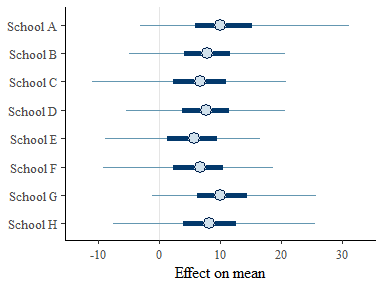Similarly important is the plot of potential treatment effects (posterior predictive distribution), obtained by repeatedly drawing hypermean and hyper-SD values from baggr’s Markov Chain model, then drawing new value of treatment effect conditional on these: effect_plot(baggr_schools)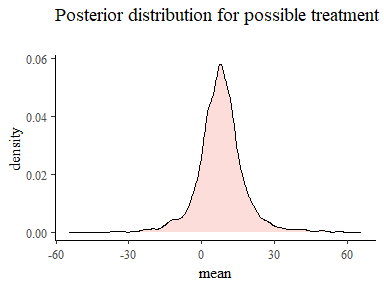The values underlying the plot can be obtained by effect_draw, which can be used e.g. to draw 1 new value: effect_draw(baggr_schools, draws = 1) #>  13.08492 You can also summarise over the entire distribution to obtain mean, SD, and quantiles (effect_draw(baggr_schools, summary = TRUE)). For meta-regression models (models with covariates), you can also use this function to make predictions for new data, at set values of covariates. However, from the viewpoint of model building, the most important plots are the ones that directly compare many possible models. ### Basic model comparison with baggr_compare The default Rubin model (which we have selected explicitly above) is that of partial pooling. When using baggr_compare without any extra arguments, full pooling, no pooling and partial pooling versions of the model will be fit: my_baggr_comparison <- baggr_compare(schools) The result of the comparison includes all the models, but it also produces an automatic comparison plot. Because the output object of baggr_compare is a ggplot object you can edit it further yourself and build on it as you would any ggplot object by, for example, changing theme or labels: plot(my_baggr_comparison) + ggtitle("8 schools: model comparison")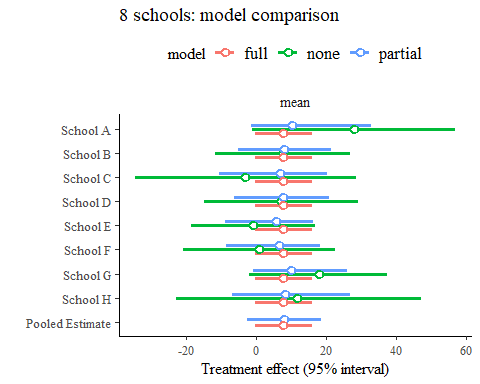### Comparing existing models, understanding impact of the prior The comparison can be made not just for different types of pooling, but for any number of models which include the same groups. A typical example is comparing two models on the same data, but with different priors. Consider this model with alternative, very strong priors: baggr_schools_v2 <- baggr(schools, prior_hypermean = normal(10, 2.5)) We can compare it with the previous model by providing both models as arguments to baggr_compare and effects_plot. It’s good to name the arguments, as these names will be used to label them: effect_plot("Default model" = baggr_schools, "normal(10, 2.5)" = baggr_schools_v2) + coord_cartesian(xlim = c(-10, 30)) + theme(legend.position = "top")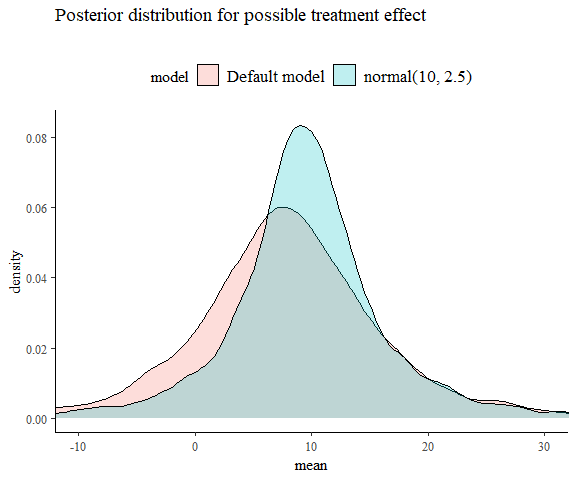baggr_compare("Default model" = baggr_schools, "normal(10, 2.5)" = baggr_schools_v2) #> #> Mean treatment effects: #> 2.5% mean 97.5% median sd #> Default model -1.88699 7.99784 18.7496 7.85416 5.32233 #> normal(10, 2.5) 5.26192 9.50358 13.7390 9.44468 2.14527 #> #> SD for treatment effects: #> 2.5% mean 97.5% median sd #> Default model 0.223366 6.42664 20.4183 5.20690 5.41337 #> normal(10, 2.5) 0.192377 5.91438 17.8139 4.88271 4.91149 #> #> Posterior predictive effects: #> 2.5% mean 97.5% median sd #> Default model -11.62300 7.98862 28.2944 7.93263 9.80165 #> normal(10, 2.5) -6.62981 9.36410 25.9881 9.25995 7.94249 ### Forest plots for the models Forest plots are a typical for reporting meta-analysis results. We adapted the default functionality from forestplot to work with baggr objects. You can choose to display 1) input data for groups, and/or, 2) posterior distributions for groups. In both cases these are followed by a display mean treatment effect. The default is 1): forest_plot(baggr_schools)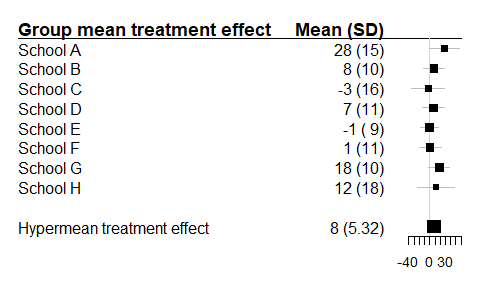The plots can be modified by passing extra arguments – see ?forestplot. It’s also possible to display both 1) and 2): forest_plot(baggr_schools, show = "both")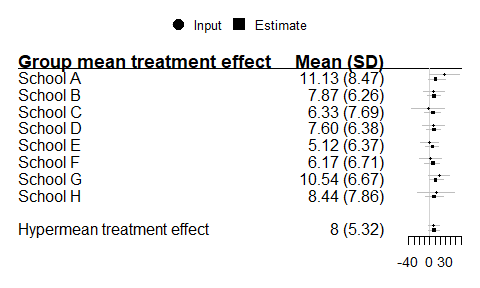## Cross-validation in baggr Baggr has built-in, automated leave-one-out cross validation for its models. The values returned by loocv() can be used to understand how any one group affects the overall result, as well as how well the model predicts the omitted group. This function automatically runs $$K$$ baggr models, leaving out one group at a time, and then calculates expected log predictive density (ELPD) for that group (see Gelman, Hwang, and Vehtari (2014)). The main output is the cross-validation information criterion, or -2 times the ELPD averaged over $$K$$ models. This is related to, and often approximated by, the Watanabe-Akaike Information Criterion. A value closer to zero (i.e. a smaller number in magnitude) means a better fit. For more information on cross-validation see this overview article. Therefore, if you have $$K$$ groups, using the loocv() will run your model of choice $$K$$ times. Be aware that this may take a while even for simple models. (You should see a progress bar in your terminal window.) The loocv() function takes in the same arguments as baggr(), plus an option (return_models) for whether to return all the models or just the summary statistics. For the 8 schools example we can do loocv_res <- loocv(schools, return_models = FALSE, iter = 1000, #just to make it a bit faster -- don't try it at home! model = "rubin", pooling = "partial") loocv_res #> LOO estimate based on 8-fold cross-validation #> #> Estimate Standard Error #> elpd -31.8 0.948 #> looic 63.5 -1.900 The loocv() output contains more than just the matrix it prints, and this additional information can be accessed via attributes(), e.g. the mean treatment effects, their variability and elpd for each model that are stored in the attribute df: names(attributes(loocv_res)) #>  "names" "class" attr(loocv_res, "df") #> NULL This data frame can then be used to examine or compute the variation in the inference on $$\tau$$ in the absence of each group. If the user is interested in manually checking the consequences of excluding a particular group or set of groups, this is also possible in baggr using subsetting. For example, suppose that we want to run the Rubin model on school groups 1-7 and predict the effect in the 8th school. The code below shows how you can specify a subset of the dataframe as your “data” argument, and then designate another subset as the “testing” holdout set by assigning it to the argument “test_data” in the baggr command. Here we have done it for both partial and full pooling: We can compare the performance of the two models using the mean log predictive density. This itself is a density as the name suggests so we will compute the log expected value of this density: here, as before, a number closer to zero is better. In this case the full pooling model actually does slightly better: fit1$mean_lpd
#>  7.934279
fit2\$mean_lpd
#>  7.739946

If we run the full loocv for both models, we can estimate the difference between the ELPD of those models as well as the standard error of that difference. As an example, let’s consider the two models above.

loocv_full <- loocv(data = schools,
model = "rubin",
pooling = "full")

We can compare those fits with loo_compare, which gives use the differences in ELPD for the various models we pass it. Generally it is important to pay attention to both the difference in the predictive density and also the standard error of that difference.

loo_compare(loocv_res, loocv_full)
#> Comparison of cross-validation
#>
#>                     ELPD ELPD SE
#> Model 1 - Model 2 -0.789   0.355